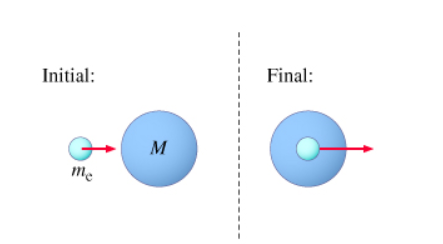# Problem: An atom of mass M is initially at rest, in its ground state. A moving (nonrelativistic) electron of mass me collides with the atom. The atom+electron system can exist in an excited state in which the electron is absorbed into the atom. The excited state has an extra, "internal," energy E relative to the atom's ground state. (Figure 1)What is the least possible initial kinetic energy Kmin the oxygen atom could have and still excite the cesium atom?

###### FREE Expert Solution

Considering that the collision is completely inelastic, the least possible kinetic energy of electron can be expressed as:

$\overline{){\mathbf{K}}{\mathbf{.}}{{\mathbf{E}}}_{\mathbf{m}\mathbf{i}\mathbf{n}}{\mathbf{=}}{\mathbf{E}}\mathbf{\left(}\frac{\mathbf{M}\mathbf{+}{\mathbf{m}}_{\mathbf{e}}}{\mathbf{M}}\mathbf{\right)}}$, where

E is the energy needed to ionize the cesium atom = 3.9 eV, M is the mass of cesium atom = 133u, and me is the mass of oxygen atom = 16u.###### Problem Details

An atom of mass M is initially at rest, in its ground state. A moving (nonrelativistic) electron of mass me collides with the atom. The atom+electron system can exist in an excited state in which the electron is absorbed into the atom. The excited state has an extra, "internal," energy E relative to the atom's ground state. (Figure 1)What is the least possible initial kinetic energy Kmin the oxygen atom could have and still excite the cesium atom?